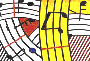OpenMusic Tutorial## Foreword

Tutorial 1 - Note transposition

Tutorial 2 - Inversion of an interval I

Tutorial 3 - Building a scale

Tutorial 4 - Inversion of an interval II

Tutorial 5 - Retrograde transposition of notes

Tutorial 6 - Four operations on a twelve-tone row

Tutorial 7 - Expansion/Compression of intervals

Tutorial 8 - Construction of a harmonic series

Tutorial 9 - Data types spectrum

Tutorial 10 - Playing MIDI

Tutorial 11 - Building a chord sequence starting from a harmonic spectrum

Tutorial 12 - Building a sequence of random notes

Tutorial 13 - Another random sequence: Introduction to durations, vels and legato

Tutorial 14 - Random construction of sequences: Introduction to omif

Tutorial 15 - Introduction to omloop I

Tutorial 16 - Introduction to omloop II

Tutorial 17 - Generation of a melodic sequence from harmonic reservoirs

Tutorial 18 - Generation of a note sequence by interpolation between two chords

Tutorial 19 - Using BPFs (I)

Tutorial 20 - Using BPFs (II)

Tutorial 21 - Using BPFs (III)

Tutorial 22 - CHORD-SEQ (I)

Tutorial 23 - CHORD-SEQ (II)

Tutorial 24 - VOICE (I)

Tutorial 25 - VOICE (II)

Tutorial 26 - Editing rhythm

Tutorial 27 - Introduction to rhythm quantification

Tutorial 28 - Working with MIDI files (I)

Tutorial 29 - Working with MIDI files (II)

Tutorial 30 - Working with Lists (I)

Tutorial 31 - Working with Lists (II)

Tutorial 32 - Introduction to the Maquette (I)

Tutorial 33 - Introduction to the Maquette (II)

Tutorial 34 - Lambda Functions (I)

Tutorial 35 - Lambda Functions (II)

Tutorial 36 - omloop: accumulation (I)

Tutorial 37 - omloop: accumulation (II)

Tutorial 38 - Recursive patch I

Tutorial 39 - Recursive patch II

Tutorial 40 - Building a maquette

Tutorial 41 -

Tutorial 42 - Creating Generic Functions (I)

Tutorial 43 - Creating Generic Functions (II)